# C Program to Multiply Two Matrices

In this article, you will learn and get code for the multiplication of two matrices in C. However, before proceeding with the program, if you are unfamiliar with how multiplication of two matrices works, I recommend that you review the step-by-step matrix multiplication process There, you can see how a multiplication of two matrices is calculated step by step using a pictorial representation. Now let's move on and implement it in a C program.

## Matrix Multiplication in C

To multiply any two matrices in C programming, first ask the user to enter any two matrices, then start multiplying the given two matrices, and store the multiplication result one by one inside any variable, say sum. Store the value of sum in the third matrix (one by one as its element), say mat3, as shown in the program given here.

The question is, "Write a program in C that multiplies two given matrices." The answer to this question is given below. This C program asks the user to enter any two 3*3 matrix elements and multiply them to form a new matrix that is the multiplication result of the two given 3*3 matrices. Here, "3*3 matrix" means a matrix that has 3 rows and 3 columns:

```#include<stdio.h>
#include<conio.h>
int main()
{
int mat1, mat2, mat3, sum=0, i, j, k;
printf("Enter first 3*3 matrix element: ");
for(i=0; i<3; i++)
{
for(j=0; j<3; j++)
scanf("%d", &mat1[i][j]);
}
printf("Enter second 3*3 matrix element: ");
for(i=0; i<3; i++)
{
for(j=0; j<3; j++)
scanf("%d", &mat2[i][j]);
}
printf("\nMultiplying two matrices...");
for(i=0; i<3; i++)
{
for(j=0; j<3; j++)
{
sum=0;
for(k=0; k<3; k++)
sum = sum + mat1[i][k] * mat2[k][j];
mat3[i][j] = sum;
}
}
printf("\nMultiplication result of the two given Matrix is: \n");
for(i=0; i<3; i++)
{
for(j=0; j<3; j++)
printf("%d\t", mat3[i][j]);
printf("\n");
}
getch();
return 0;
}```

As the above program was written in the Code::Blocks IDE, here is the output you will see on your screen after a successful build and run. This is the first snapshot: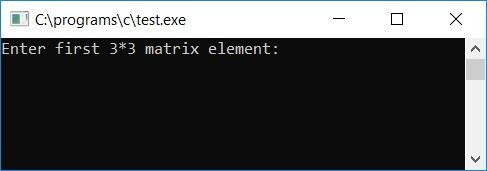Now, for the first 3*3 matrix, provide any 9 elements, followed by another 9 elements for the second 3*3 matrix. After you've entered all of the 9-9 elements for both the 3*3 matrix, press ENTER to see the multiplication result of the two matrices, as shown in the second screenshot of the sample run below: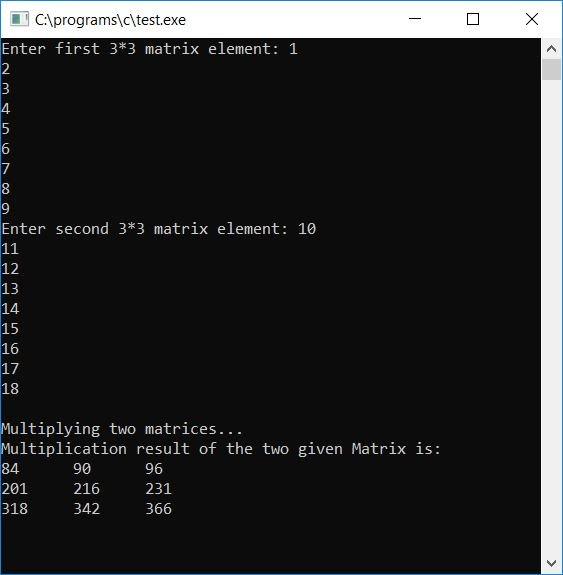#### Program Explained

1. Get the first nine elements, or numbers, from the user and store them inside the first matrix, index-wise, from 00 to 22.
2. That is, the first element stored inside mat1, the second element stored inside mat1, the third element stored inside mat1, ... the seventh element stored inside mat1, the eighth element stored inside mat1, and the last or ninth element stored inside mat1.
3. In a similar way, get the second set of 9 elements from the user and store it inside the second matrix.
4. We now have two 3*3 matrices with 9-9 elements in each.
5. Here, we've used three loops to multiply the matrices. The first two are used for row and column, while the third is used to apply the matrix's multiplication rule.
6. Apply the matrix multiplication rule to the matrix and multiply it; once the result is obtained, store the value inside the sum variable after multiplying each row element of the first matrix by the corresponding column element of the second matrix, and one by one initialize the value of the sum variable into the third matrix.Never forget to initialize 0 to sum before the multiplying process starts for each index of the third matrix.
7. In this way, the third matrix, say mat3, contains a total of 9 elements that will be the multiplication result of the two given matrices, say mat1 and mat2.
8. Finally, print the value of the third matrix.

### Allow the user to specify the size of the matrix

Now let's modify the above program by implementing an extra feature. That is, this program allows the user to define the size of the matrix.

```#include<stdio.h>
#include<conio.h>
int main()
{
int mat1, mat2, matmult;
int row1, col1, row2, col2, i, j, k, sum;
printf("Enter size of first matrix:\n");
printf("Enter row size: ");
scanf("%d", &row1);
printf("Enter column size: ");
scanf("%d", &col1);
printf("\nEnter the element of first matrix:\n");
for(i=0; i<row1; i++)
{
for(j=0; j<col1; j++)
scanf("%d", &mat1[i][j]);
}
printf("\nEnter size of second matrix:\n");
printf("Enter row size: ");
scanf("%d", &row2);
printf("Enter column size: ");
scanf("%d", &col2);
printf("\nEnter the element of second matrix:\n");
for(i=0; i<row2; i++)
{
for(j=0; j<col2; j++)
scanf("%d", &mat2[i][j]);
}
if(col1!=row2)
{
printf("\nMultiplication not possible!");
printf("\nExiting...\n");
printf("Press any key...");
getch();
return 0;
}
printf("\nMultiplying the two matrix...\n");
for(i=0; i<row1; i++)
{
for(j=0; j<col2; j++)
{
sum = 0;
for(k=0; k<col1; k++)
sum = sum + mat1[i][k] * mat2[k][j];
matmult[i][j] = sum;
}
}
printf("The multiplication result (resultant matrix) is:\n");
for(i=0; i<row1; i++)
{
for(j=0; j<col2; j++)
printf("%d ", matmult[i][j]);
printf("\n");
}
getch();
return 0;
}```

Before multiplying the two given matrices entered at run-time, we have applied an if statement to check whether the column size of the first matrices is equal to the row size of the second matrices or not.

If it is equal, then start multiplying and find out the result. If it isn't, print something like "Multiplication not possible!" Here is the sample run: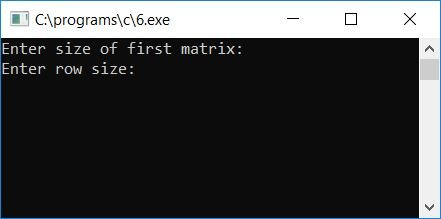The following is a snapshot after providing row and column sizes as well as matrix elements (for the first matrix):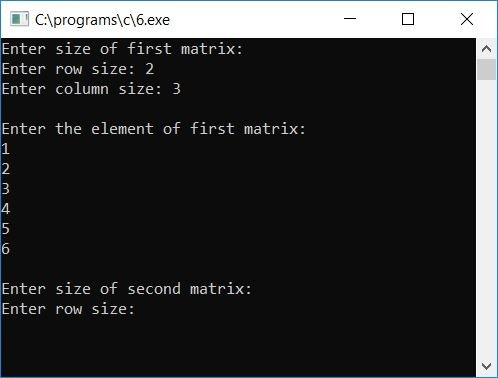The snapshot after providing row and column sizes along with matrix elements (for the second matrix). Because we have provided 3 as the row size of the second matrix, which is equal to the column size of the first matrix:Now let's take another sample run. In this case, let's suppose the user has entered the column size of the first matrix as 3 and the row size of the second matrix as 2, which are not equal: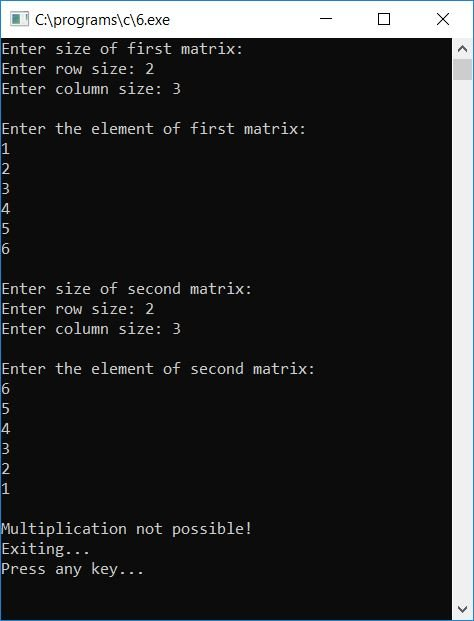As you can clearly see from the above sample runs, matrix multiplication is not possible if the column size of the first matrix is not equal to the row size of the second matrix.

#### The same program in different languages

C Quiz

« Previous Program Next Program »